# Modify `surrogateopt` Options

This example shows how to search for a global minimum by running `surrogateopt` on a two-dimensional problem that has six local minima. The example then shows how to modify some options to search more effectively.

Define the objective function `sixmin` as follows.

```sixmin = @(x)(4*x(:,1).^2 - 2.1*x(:,1).^4 + x(:,1).^6/3 ... + x(:,1).*x(:,2) - 4*x(:,2).^2 + 4*x(:,2).^4);```

Plot the function.

```[X,Y] = meshgrid(linspace(-2.1,2.1),linspace(-1.2,1.2)); Z = sixmin([X(:),Y(:)]); Z = reshape(Z,size(X)); surf(X,Y,Z,'EdgeColor','none') view(-139,31)```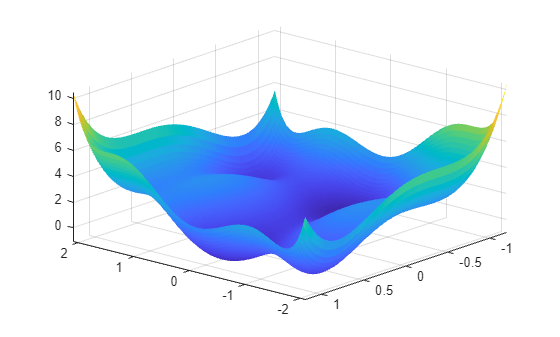The function has six local minima and two global minima.

Run `surrogateopt` on the problem using the `'surrogateoptplot'` plot function in the region bounded in each direction by `[-2.1,2.1]`. To understand the `'surrogateoptplot'` plot, see Interpret surrogateoptplot.

```rng default lb = [-2.1,-2.1]; ub = -lb; opts = optimoptions('surrogateopt','PlotFcn','surrogateoptplot'); [xs,fvals,eflags,outputs] = surrogateopt(sixmin,lb,ub,opts);```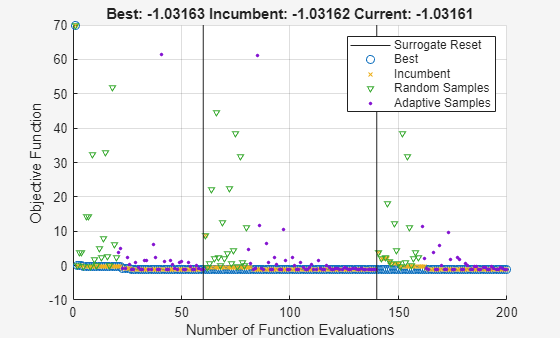```Surrogateopt stopped because it exceeded the function evaluation limit set by 'options.MaxFunctionEvaluations'. ```

Set a smaller value for the `MinSurrogatePoints` option to see whether the change helps the solver reach the global minimum faster.

```opts.MinSurrogatePoints = 4; [xs2,fvals2,eflags2,outputs2] = surrogateopt(sixmin,lb,ub,opts);```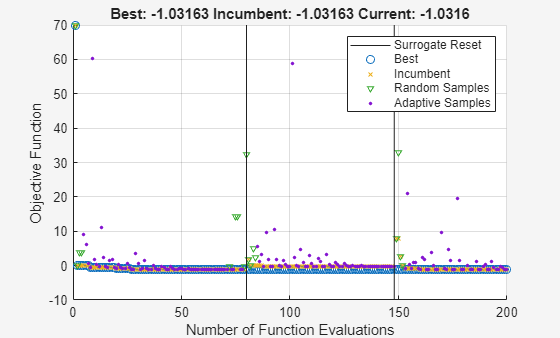```Surrogateopt stopped because it exceeded the function evaluation limit set by 'options.MaxFunctionEvaluations'. ```

The smaller `MinSurrogatePoints` option does not noticeably change the solver behavior.

Try setting a larger value of the `MinSampleDistance` option.

```opts.MinSampleDistance = 0.05; [xs3,fvals3,eflags3,outputs3] = surrogateopt(sixmin,lb,ub,opts);```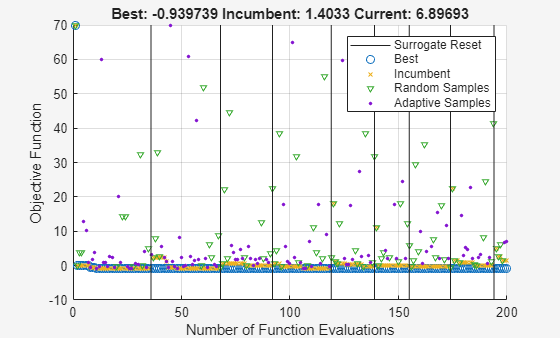```Surrogateopt stopped because it exceeded the function evaluation limit set by 'options.MaxFunctionEvaluations'. ```

Changing the `MinSampleDistance` option has a small effect on the solver. This setting causes the surrogate to reset more often, and causes the best objective function to be slightly higher (worse) than before.

Try using parallel processing. Time the execution both with and without parallel processing on the `camelback` function, which is a variant of the `sixmin` function. To simulate a time-consuming function, the `camelback` function has an added pause of one second for each function evaluation.

`type camelback`
```function y = camelback(x) y = (4*x(1)^2 - 2.1*x(1)^4 + x(1)^6/3 ... + x(1)*x(2) - 4*x(2)^2 + 4*x(2)^4); pause(1) ```
```tic opts = optimoptions('surrogateopt','UseParallel',true,'PlotFcn','surrogateoptplot'); [xs4,fvals4,eflags4,outputs4] = surrogateopt(@camelback,lb,ub,opts);```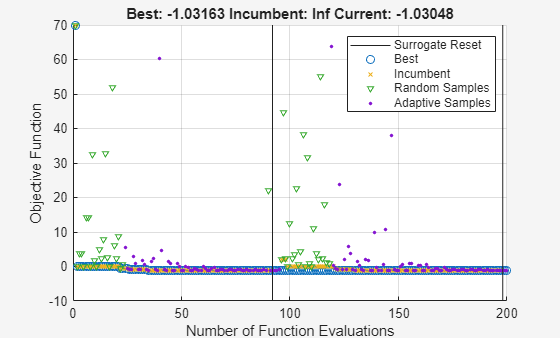```Surrogateopt stopped because it exceeded the function evaluation limit set by 'options.MaxFunctionEvaluations'. ```
`toc`
```Elapsed time is 43.142697 seconds. ```

Time the solver when run on the same problem in serial.

```opts.UseParallel = false; tic [xs5,fvals5,eflags5,outputs5] = surrogateopt(@camelback,lb,ub,opts);```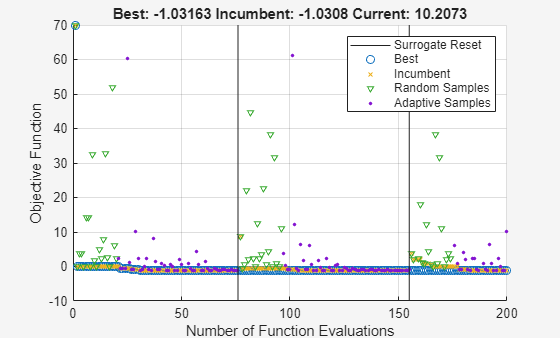```Surrogateopt stopped because it exceeded the function evaluation limit set by 'options.MaxFunctionEvaluations'. ```
`toc`
```Elapsed time is 227.968689 seconds. ```

For time-consuming objective functions, parallel processing significantly improves the speed, without overly affecting the results.